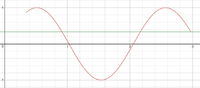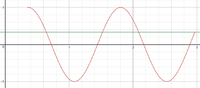# Trigonometric equation

#### Sweden_01

##### New member
Hi!

I am really stuck on this problem (below) and would be extremely thankful for some help.

The problem: The equation 3*sin(k(x−10)) = 1 has 3 solutions on the interval 30°≤ X ≥ 180°. Determine/calculate all possible positive values of the constant k.

I have a math test coming up soon and would really appreciate some help. Thanks in advance.

#### Subhotosh Khan

##### Super Moderator
Staff member
Hi!

I am really stuck on this problem (below) and would be extremely thankful for some help.

The problem: The equation 3*sin(k(x−10)) = 1 has 3 solutions on the interval 30°≤ X ≥ 180°. Determine/calculate all possible positive values of the constant k.

I have a math test coming up soon and would really appreciate some help. Thanks in advance.
Is 'x' = 'X'

Yes, it is.

#### Subhotosh Khan

##### Super Moderator
Staff member
Is 'x' = 'X'
Hi!

I am really stuck on this problem (below) and would be extremely thankful for some help.

The problem: The equation 3*sin(k(x−10)) = 1 has 3 solutions on the interval 30°≤ X ≥ 180°. Determine/calculate all possible positive values of the constant k.

I have a math test coming up soon and would really appreciate some help. Thanks in advance.
Is that domain stated correctly?

#### Sweden_01

##### New member
Ooopps. It is supposed to be 30°≤ X ≤ 180°.

Honestly, I don't know where to begin. I've been doing trigonometry a good amount of time, however, this problem is really unclear to me. I do know how to solve problems where I am supposed to give all the solutions, such as 3sin(kx) = 0.8. However, I haven't encountered this kind of problem (the first one) before and would really appreciate some help/guidance.

#### MarkFL

##### Super Moderator
Staff member
The parameter $$k$$ is going to affect two aspects of the sine function on the LHS of the equation: the period and the phase shift. I think I would begin by eliminating the effect on the phase shift by stating:

$$\displaystyle \sin(k\theta)=\frac{1}{3}$$ where $$20^{\circ}\le\theta\le170^{\circ}$$

What is the period of this sine function?

#### Sweden_01

##### New member
The period P should be: P = 2π/k

•MarkFL

#### MarkFL

##### Super Moderator
Staff member
The period P should be: P = 2π/k
Yes, good!We see that our domain spans $$150^{\circ}$$. In one period of a sine function, how many times does it reach some positive value?

#### Sweden_01

##### New member
Well, in one period it's under zero one time. But it reaches the positive values to times, so first positive then negative then positive again.

#### Jomo

##### Elite Member
Yes, it is.
Then either state that x=X (by writing x=X) or just use x OR X.

#### Sweden_01

##### New member
Then either state that x=X (by writing x=X) or just use x OR X.
Will try to do it in future questions, in order to be clear.

#### MarkFL

##### Super Moderator
Staff member
Yes, in one period the sine function will take on a particular positive value (within the range) two times. So, this tells us the period must be smaller than $$170^{\circ}$$:

$$\displaystyle \frac{360^{\circ}}{k}<170^{\circ}$$

$$\displaystyle \frac{36}{k}<15$$

$$\displaystyle \frac{36}{17}<k$$

Do we find the following?

$$\displaystyle \sin\left(\frac{36}{17}20^{\circ}\right)>\frac{1}{3}$$

•topsquark

#### Sweden_01

##### New member
So the last inequality, LHS is greater than RHS is obviously correct. But where did 20° come from?

#### MarkFL

##### Super Moderator
Staff member
That's the lower bound of our new domain, on $$\theta$$.

Okay, given that this inequality is true, then that means the sine function starts out above the value we want, and so we must look for when it is decreasing, and then an increase and another decrease, like this:So, we need:

$$\displaystyle \sin(k\cdot170^{\circ})=\frac{1}{3}$$

What is the smallest value of $$k$$, given the restriction on $$k$$ we already determined, that satisfies the above?

•topsquark

#### Sweden_01

##### New member
Well, the smallest positive value of k should be:

k*170 = arcsin (1/3)

(1): k ≈ 0,11 + n*(360/170)

(2): k ≈ 0,94 + n*(360/170)

Hence the smallest positive value of k should be ≈ 0,11

#### MarkFL

##### Super Moderator
Staff member
I get:

$$\displaystyle k=\frac{18\left(3\pi-\arcsin\left(\dfrac{1}{3}\right)\right)}{17\pi}\approx3.0619339962677$$

Since we're catching it while decreasing a second time, we want:

$$\displaystyle \sin\left(\pi-k\left(\frac{17\pi}{18}-1\cdot\frac{2\pi}{k}\right)\right)=\sin\left(3\pi-k\frac{17\pi}{18}\right)=\frac{1}{3}$$

$$\displaystyle 3\pi-k\frac{17\pi}{18}=\arcsin\left(\frac{1}{3}\right)$$

And as you can see, this leads to the value above, which is the lower bound (inclusive) on $$k$$

To determine the upper bound, we need to catch the sine function on the rise for the third time:$$\displaystyle \sin\left(k\left(\frac{17\pi}{18}-2\cdot\frac{2\pi}{k}\right)\right)=\sin\left(k\frac{17\pi}{18}-4\pi\right)=\frac{1}{3}$$

What do you get for $$k$$ here?

Last edited:
•topsquark and Sweden_01

#### Sweden_01

##### New member
To be honest, I don't really understand how k ≈ 3.0619339962677. I would really appreciate it if you could explain that step (I know I am a bit slow in math, well trigonometry isn't my strongest side) but the help I am receiving here is invaluable.

#### MarkFL

##### Super Moderator
Staff member
What I utilized there is the identity:

$$\displaystyle \sin(x)=\sin(\pi-x)$$

This takes care of the decreasing aspect. And then to catch the second decrease, we need to consider the periodic nature of the sine function:

$$\displaystyle \sin(x)=\sin(x+2\pi)$$

And then combining the two, we have:

$$\displaystyle \sin(x)=\sin(\pi-x+2\pi)=\sin(3\pi-x)$$

And we then use (converting degrees to radians):

$$\displaystyle x=k\cdot170^{\circ}=k\frac{17\pi}{18}$$

Thus:

$$\displaystyle \sin\left(3\pi-k\frac{17\pi}{18}\right)=\frac{1}{3}$$

Does that make sense? Sorry, I have to run now, but hopefully you see how the upper bound equation was determined as well.•Sweden_01

#### Sweden_01

##### New member
Yep, it made a lot of things clear now, thanks for all the time you put down helping me. Now I will try to solve this with the information we concluded and, if still having a problem, will ask for more help. Have a nice evening/day (depends on where you live) and thanks again for all the effort you put down in helping me!

•MarkFL

#### MarkFL

##### Super Moderator
Staff member
I edited post #16 to add some clarity and to correct an error I made.

In the end, you should get for $$k$$, the interval:

$$\displaystyle \left[\frac{18\left(3\pi-\arcsin\left(\dfrac{1}{3}\right)\right)}{17\pi},\frac{18\left(4\pi+\arcsin\left(\dfrac{1}{3}\right)\right)}{17\pi}\right)$$

•Sweden_01 and topsquark